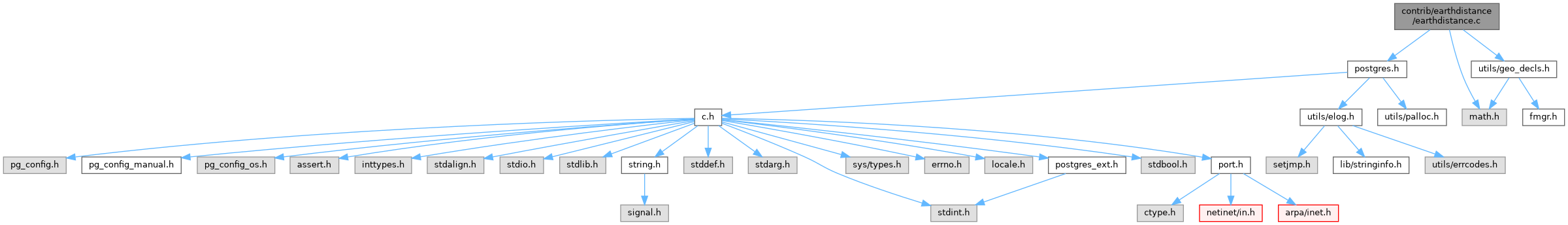PostgreSQL Source Code  git master
earthdistance.c File Reference
`#include "postgres.h"`
`#include <math.h>`
`#include "utils/geo_decls.h"`
Include dependency graph for earthdistance.c:Go to the source code of this file.

## Macros

#define M_PI   3.14159265358979323846

## Functions

static double geo_distance_internal (Point *pt1, Point *pt2)

PG_FUNCTION_INFO_V1 (geo_distance)

Datum geo_distance (PG_FUNCTION_ARGS)

## Variables

PG_MODULE_MAGIC

static const double EARTH_RADIUS = 3958.747716

static const double TWO_PI = 2.0 * M_PI

## ◆ M_PI

 #define M_PI   3.14159265358979323846

Definition at line 11 of file earthdistance.c.

## Function Documentation

 static double degtorad ( double degrees )
static

Definition at line 31 of file earthdistance.c.

32 {
33  return (degrees / 360.0) * TWO_PI;
34 }
static const double TWO_PI
Definition: earthdistance.c:18
Datum degrees(PG_FUNCTION_ARGS)
Definition: float.c:2560

References degrees(), and TWO_PI.

Referenced by geo_distance_internal().

## ◆ geo_distance()

 Datum geo_distance ( PG_FUNCTION_ARGS )

Definition at line 97 of file earthdistance.c.

98 {
99  Point *pt1 = PG_GETARG_POINT_P(0);
100  Point *pt2 = PG_GETARG_POINT_P(1);
101  float8 result;
102
103  result = geo_distance_internal(pt1, pt2);
104  PG_RETURN_FLOAT8(result);
105 }
double float8
Definition: c.h:614
static double geo_distance_internal(Point *pt1, Point *pt2)
Definition: earthdistance.c:50
#define PG_RETURN_FLOAT8(x)
Definition: fmgr.h:367
#define PG_GETARG_POINT_P(n)
Definition: geo_decls.h:185

References geo_distance_internal(), PG_GETARG_POINT_P, and PG_RETURN_FLOAT8.

## ◆ geo_distance_internal()

 static double geo_distance_internal ( Point * pt1, Point * pt2 )
static

Definition at line 50 of file earthdistance.c.

51 {
52  double long1,
53  lat1,
54  long2,
55  lat2;
56  double longdiff;
57  double sino;
58
59  /* convert degrees to radians */
60
63
66
67  /* compute difference in longitudes - want < 180 degrees */
68  longdiff = fabs(long1 - long2);
69  if (longdiff > M_PI)
70  longdiff = TWO_PI - longdiff;
71
72  sino = sqrt(sin(fabs(lat1 - lat2) / 2.) * sin(fabs(lat1 - lat2) / 2.) +
73  cos(lat1) * cos(lat2) * sin(longdiff / 2.) * sin(longdiff / 2.));
74  if (sino > 1.)
75  sino = 1.;
76
77  return 2. * EARTH_RADIUS * asin(sino);
78 }
Definition: earthdistance.c:31
Definition: earthdistance.c:17
#define M_PI
Definition: earthdistance.c:11
float8 y
Definition: geo_decls.h:99
float8 x
Definition: geo_decls.h:98

Referenced by geo_distance().

## ◆ PG_FUNCTION_INFO_V1()

 PG_FUNCTION_INFO_V1 ( geo_distance )

## Variable Documentation

static

Definition at line 17 of file earthdistance.c.

Referenced by geo_distance_internal().

## ◆ PG_MODULE_MAGIC

 PG_MODULE_MAGIC

Definition at line 14 of file earthdistance.c.

## ◆ TWO_PI

 const double TWO_PI = 2.0 * M_PI
static

Definition at line 18 of file earthdistance.c.